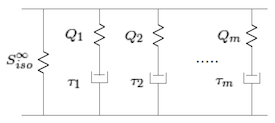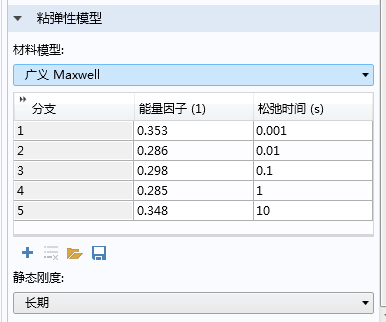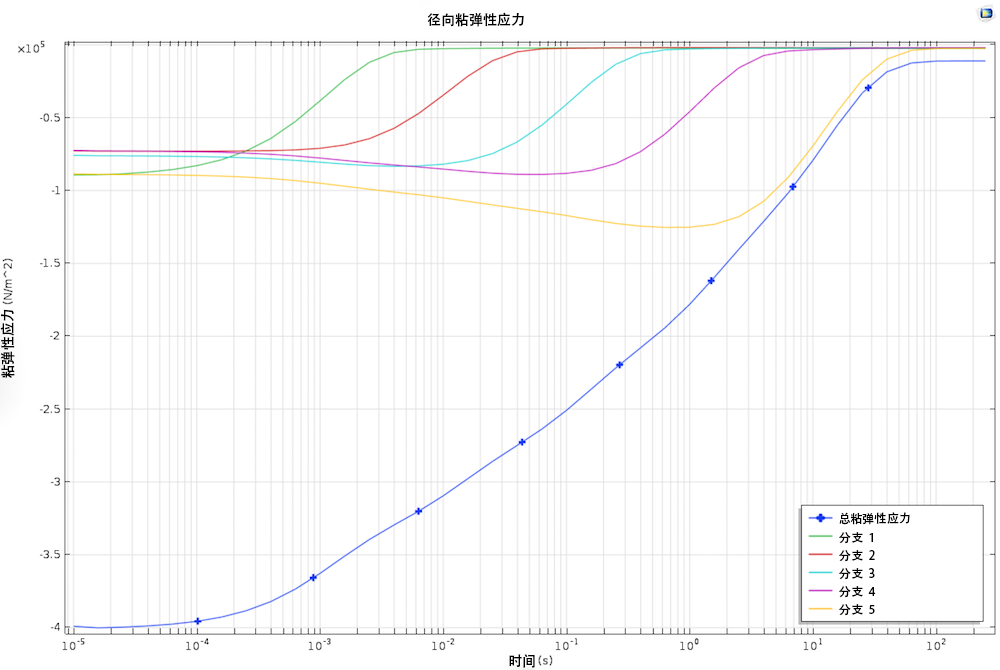# 如何在 COMSOL Multiphysics® 中模拟大应变粘弹性

2016年 10月 11日

### 动脉的力学性能### 大应变粘弹性的理论

Bower 在《应用固体力学》一书中指出：“相比于有限应变塑性理论，有限应变粘弹性理论还不成熟，目前尚没有一个统一的公式对其进行描述。”COMSOL Multiphysics 5.2a 版本为用户提供了 Holzapfel 模型，它不仅可用于模拟大应变粘弹性（参考文献 1参考文献 3），而且十分适合与 COMSOL® 软件中的任意预定义超弹性材料模型进行耦合。

W(C,\Gamma_m) = W_{vol}(J) + W_{iso}(\bar{C}) + \sum_{m} \Psi_m(\bar{C},\Gamma_m)

S = 2\frac{\partial W}{\partial C} = S_{vol}^\infty + S_{iso}^\infty + 2\sum_m \frac{\partial \Psi_m}{\partial C}

Q_m =2 \frac{\partial \Psi_m(\bar{C},\Gamma_m)}{\partial C} =-2 \frac{\partial \Psi_m(\bar{C},\Gamma_m)}{\partial \Gamma_m}

S = 2\frac{\partial W}{\partial C} = S_{vol}^\infty + S_{iso}^\infty + \sum_m Q_m\dot{Q}_m+\frac{1}{\tau_m}Q_m = \dot{S}_{iso,m}

Holzapfel 模型还假设在每个分支上都具有与弹性相关的等体积应变能量密度
W_{iso, m}(\bar{C})，由此得出

S_{iso, m} = 2\frac{\partial W_{iso,m}}{\partial C}

Holzapfel 形式中最主要的假设是：每一个分支的等体积应变能均取决于主超弹性分支的等体积应变能

W_{iso, m}= \beta_\alpha W_{iso}^\infty(\bar{C})

S_{iso, m} = 2\frac{\partial W_{iso,m}}{\partial C} = \beta_mS_{iso}^\infty

\dot{Q}_m+\frac{1}{\tau_m}Q_m = \beta_m \dot{S}_{iso}^\infty### 在 COMSOL Multiphysics 中模拟大应变粘弹性### 参考文献

1. G. Holzapfel, Nonlinear Solid Mechanics: A Continuum Approach for Engineering, John Wiley & Sons, 2000.
2. G. Holzapfel, T. Gasser, and R. Ogden, “A New Constitutive Framework for Arterial Wall Mechanics and a Comparative Study of Material Models,” J. Elasticity, vol. 61, pp. 1–48, 2000.
3. G. Holzapfel, T. Gasser, and M. Stadler, “A Structural Model for the Viscoelastic Behavior of Arterial Walls: Continuum Formulation and Finite Element Analysis”, European Journal of Mechanics A/Solid, vol.21, pp. 441–463, 2002.

#### 评论 (2)

##### 留言##### 建华 金
2017-06-06

good2017-06-06